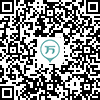150:002017年成人高考（专起点）《大学语文》真题
1～20小题，每小题2分，共40分。在每小题给出的四个选项中，只有一项是符合题目要求的，把所选项前的字母填在题后的括号内。
1
(单项选择题)

• A.

五言古诗

• B.

五言律诗

• C.

五言绝句

• D.

杂言诗

• A
• B
• C
• D

2
(单项选择题)

• A.

闽东风情

• B.

湘西风情

• C.

赣南风情

• D.

北平风情

• A
• B
• C
• D

3
(单项选择题)

• A.

曹操

• B.

陶渊明

• C.

李白

• D.

王维

• A
• B
• C
• D

4
(单项选择题)

• A.

李白《行路难》、杜甫《蜀相》

• B.

陆游《关山月》、李白《行路难》

• C.

曹操《短歌行》、陶渊明《饮酒》

• D.

王昌龄《从军行》、白居易《杜陵叟》

• A
• B
• C
• D

5
(单项选择题)

• A.

《张中丞传后叙》《陈情表》

• B.

《五代史伶官传序》《马伶传》

• C.

《李将军列传》《冯谖客孟尝君》

• D.

《李将军列传》《马伶传》

• A
• B
• C
• D

6
(单项选择题)

《苦恼》《猎人笔记》《麦琪的礼物》的作者依次是（　）。

• A.

屠格涅夫、欧·亨利、契诃夫

• B.

契诃夫、屠格涅夫、欧·亨利

• C.

欧·亨利、契诃夫、屠格涅夫

• D.

契诃夫、欧·亨利、屠格涅夫

• A
• B
• C
• D

7
(单项选择题)

• A.

《报刘一丈书》－侯方域－书信体议论文

• B.

《论毅力》－梁实秋－议论文

• C.

《答司马谏议书》－王安石－书信体驳论文

• D.

《陈情表》－李斯－奏章

• A
• B
• C
• D

8
(单项选择题)

• A.

是一篇文赋

• B.

文章的语言骈散相同，音韵和谐

• C.

文章在结构上采用的是主客问答、伸客抑主的方法

• D.

文章中的景物描写体现出作者乐观豁达的人生态度

• A
• B
• C
• D

9
(单项选择题)

• A.

是一首汉乐府民歌

• B.

是一首叙事诗

• C.

诗中主人公是罗敷

• D.

是一首表达青年男女爱情的诗歌

• A
• B
• C
• D

10
(单项选择题)

• A.

曹禺《日出》

• B.

郁达夫《故都的秋》

• C.

茅盾《香市》

• D.

鲁迅《灯下漫笔》

• A
• B
• C
• D

11
(单项选择题)

• A.

文学研究会、新月社、创造社

• B.

创造社、文学研究会、新月社

• C.

文学研究会、创造社、新月社

• D.

新月社、文学研究会、创造社

• A
• B
• C
• D

12
(单项选择题)

• A.

词起始于唐代，盛行于宋代

• B.

词由五言诗、七言诗和民间歌谣发展而成

• C.

词有词牌，不同词牌有不同的押韵规则

• D.

词可分为“婉约”与“豪放”两大流派，并以“豪放派”为正宗

• A
• B
• C
• D

13
(单项选择题)

“天下为公”“狡兔三窟”两个成语依次出自（　）。

• A.

《礼记·大同》《冯谖客孟尝君》

• B.

《季氏将伐颛臾》《李将军列传》

• C.

《冯谖客孟尝君》《季氏将伐颛臾》

• D.

《李将军列传》《礼记·大同》

• A
• B
• C
• D

14
(单项选择题)

• A.

“初唐四杰”是指王勃、杨炯、卢照邻、骆宾王

• B.

“中唐古文运动”的领袖是韩愈、柳宗元

• C.

“新乐府运动”的倡导者是王维、白居易

• D.

“小李杜”指的是李商隐、杜牧

• A
• B
• C
• D

15
(单项选择题)

• A.

恨相见得迟，怨归去得疾。

• B.

泪添九曲黄河溢，恨压三峰华岳低

• C.

晓镜但愁云鬓改，夜吟应觉月光寒

• D.

忽如一夜春风来，千树万树梨花开

• A
• B
• C
• D

16
(单项选择题)

• A.

襟袖红泪：相传魏文帝时，薛灵云被选入宫，泣别父母，以玉壶盛泪，不久泪凝如血。

• B.

树犹如此：相传桓温北伐路过金城，看到多年前自己种的柳树已有十围粗了，感叹地说：“木犹如此，人何以堪！”

• C.

周公吐哺：相传周公唯恐失天下之士，常常吃饭时停下来接待贤才。

• D.

乘舟梦日：相传吕尚在被文王征聘前，曾梦见自己乘船从日月旁经过。

• A
• B
• C
• D

17
(单项选择题)

• A.

左右曰：“乃歌夫‘长铗归来’者也。”

• B.

梁惠王曰：“寡人之于国也，尽心焉耳矣！……”

• C.

问者嘻曰：“不亦善夫！吾问养树，得养人术。”

• D.

每大言曰：“人生有命，吾惟守分尔！”

• A
• B
• C
• D

18
(单项选择题)

• A.

井蛙不可以语于海者，[拘于虚也]。

拘于虚也：被狭小的居处所局限。

• B.

抽矢射佛寺浮图，[矢著其上砖半箭]。

矢著其上砖半箭：箭身一半射进佛塔上边的砖中。

• C.

欲与大叔，[臣请事之]。

臣请事之：请允许我去侍奉他。

• D.

危而不持，颠而不扶，[则将焉用彼相矣]？

则将焉用彼相矣：将怎么用你们当丞相呢？

• A
• B
• C
• D

19
(单项选择题)

• A.

三【顾】频烦天下计

顾：回头看

• B.

麦苗不【秀】多黄死

秀：开花

• C.

黄沙百战【穿】金甲

穿：穿上

• D.

狐裘不暖锦【衾】薄

衾：衣服

• A
• B
• C
• D

20
(单项选择题)

• A.

外无待而犹死守，人相食且尽。

• B.

岂吾相不当侯邪？且固命也？

• C.

且臣结发而与匈奴战，今乃一得当单于。

• D.

虽食，且不下咽。

• A
• B
• C
• D